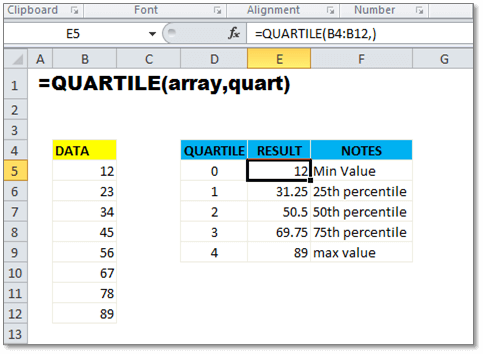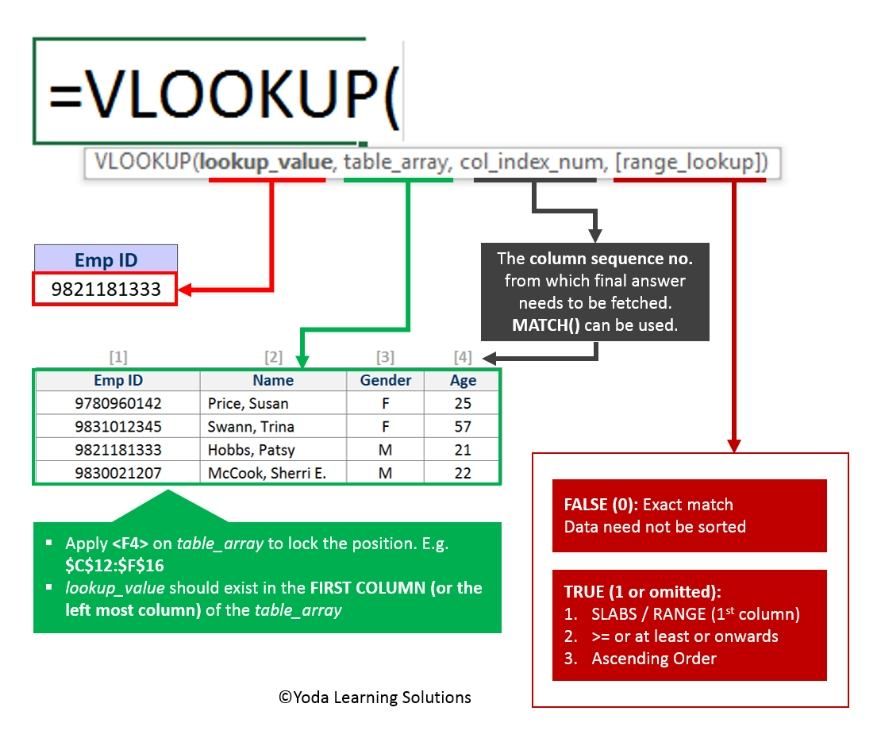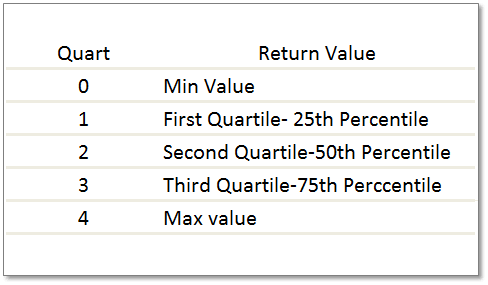# Quartile Function in Excel

The Quartile Function in Excel helps to return the quartile of a data set in Excel. Going further in this tutorial, you’ll see how to find Quartile using Excel. You’ll observe that the Quartiles are several times used in sales and also while inspecting the sample data.The Quartile Function in Excel will support you while dividing the population into groups. For instance, let’s consider an example, you can even use the QUARTILE function in Excel to discover the income in a population, i.e. top 25 percent of incomes in a population.

The Excel QUARTILE function helps to return the quartile, individually from the four equal groups. This is for a data-set. The QUARTILE Function will help to return the minimum value, first quartile, second quartile, third quartile, and then the max value.

Excel Vlookup formula – GuidebookIf Yes, you will enjoy this guidebook on ‘Excel Vlookup Formulas’ – VLOOKUP, HLOOKUP, MATCH & INDEX.

### Purpose

You want the quartile present in a data set

### Return value

You need to know the Value requested for the quartile

### Syntax

=QUARTILE (array, quart)

### Arguments

array – A reference that contains the data for analyzing.

quart – The quartile value to be returned.

## What is Quartile in Excel?

Quartile function in Excel. The Quartile function is a type of quantile (in statistics). The three points that will divide the sorted Datasets into Four groups (the groups are equal). Each of these represents a fourth of the distributed sample Population.

### There are three Quartiles while you talk about the Quartile Function:

• The first quartile also called (Q1)
• The second quartile also called (Q2)
• The third quartile also called (Q3)

You can even check out our blog on R1C1 Reference Style which we uploaded last time.

## How to Use Quartile Function in Excel

To use quartile function in excel, you should follow the below steps,

1. The Quartile Function in Excel returns the Quartile (each of four equal groups) for a given dataset.
2. Get a quartile function in a set of data
3. Return Value for requested quartile
4. Use syntax =QUARTILE (array, quart)
array – A reference containing data to analyze
quart – The return value of quartile
5. Use the QUARTILE function to get the quartile for a given set of data.

15 Excel Data Cleaning Tricks Guidebook

Bored of downloading text heavy / copy-pasted eBooks? If Yes, you will enjoy this guidebook (43 pages)

### Practice Notes

The QUARTILE function in Excel is to be used for a set of Data to get the quartile. QUARTILE contains two arguments, the array, and the quart. The array contains the numeric data which will help you to analyze and quart which indicates which quartile value is to be returned back. 5 values are displayed for the quart argument. Here, you’ll be able to see how the QUARTILE function accepts these values.Important: The quartile Function has been replaced by one or more new Functions. These functions propose enhanced accuracy in results. And the names of these already reflect on their usage. The quartile function is made available to date. But this used to be helpful for the Backward Compatibility. Since there might be no availability of the quartile function in the Future. Therefore, people should not rely on these Functions anymore.

Excel Vlookup formula – GuidebookIf Yes, you will enjoy this guidebook on ‘Excel Vlookup Formulas’ – VLOOKUP, HLOOKUP, MATCH & INDEX.

## How to find Quartiles Function in Excel

To find the Quartiles of this data set in Excel, follow these steps:

1. Follow the order of the data from least to greatest
2. Divide the data set into two halves after you discover the median
3. Now discover the median of the two halves